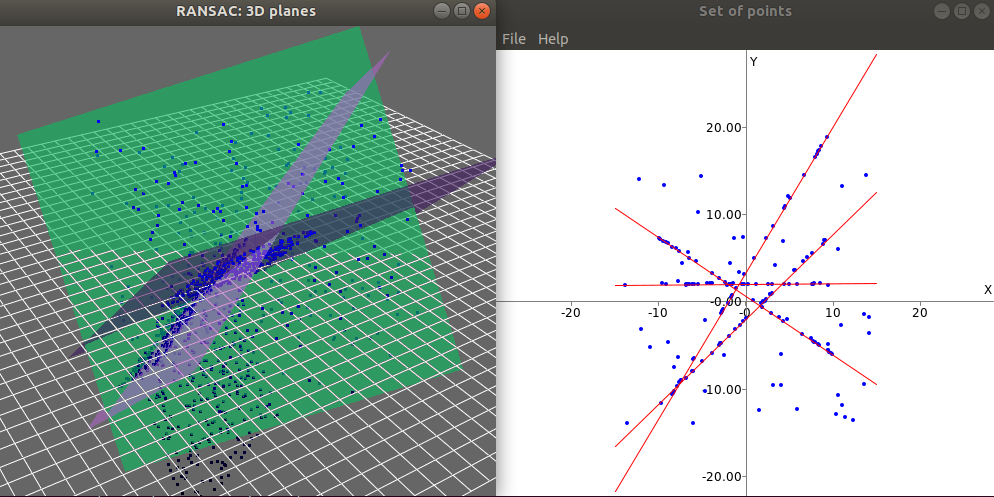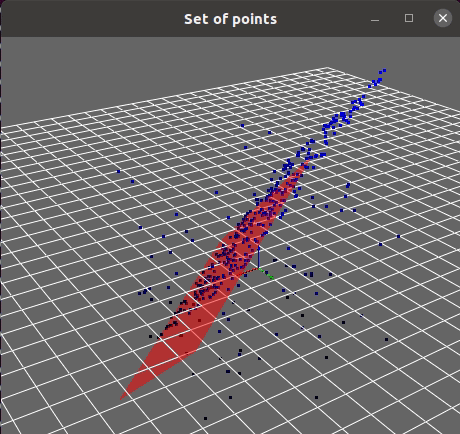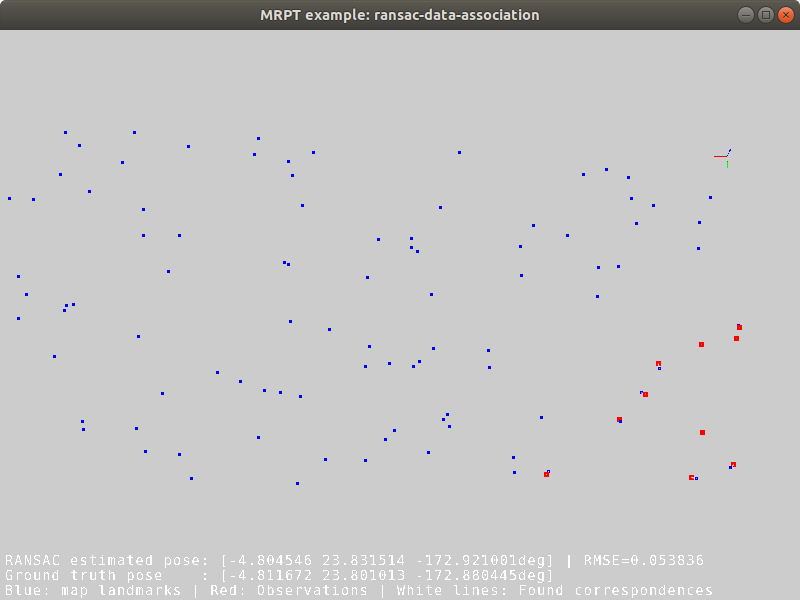# RANSAC C++ examples

## 1. RANSAC algorithm

Random sample consensus (RANSAC) was originally presented in the seminal work [FB81] and is still widely used nowadays (e.g. in the front-end of Visual SLAM or Visual Odometry systems). For a theoretical description of the algorithm, refer to [FB81] or to this Wikipedia article and the cites herein.

MRPT comprises a generic, template-based C++ implementation of this robust model fit algorithm, useful for outliers rejection. See also this excellent MATLAB toolkit by Peter Kovesi, on which MRPT implementation is strongly based.## 2. C++ API

The base C++ API for RANSAC in MRPT is mrpt::math::RANSAC_Template, while some specialized classes exist for particular instances of common problems, e.g. fit a plane or a line to a point cloud, as shown below.

A simple genetic-like modification of RANSAC is also available through the template class mrpt::math::ModelSearch.

## 3. Particular applications

### 3.1. Fit a plane from 3D points

Refer to the example source code for a direct usage of the generic C++ RANSAC template to see how to define custom models and test functions.### 3.2. Fit many planes from 3D points

MRPT provides the following functions for detecting planes (see their C++ API documentation or the complete example):

Fit planes C++ API
```template <typename NUMTYPE>
void mrpt::math::ransac_detect_3D_planes(
const CVectorDynamic<NUMTYPE>& x,
const CVectorDynamic<NUMTYPE>& y,
const CVectorDynamic<NUMTYPE>& z,
std::vector<std::pair<size_t, TPlane>>& out_detected_planes,
const double threshold,
const size_t min_inliers_for_valid_plane = 10
)

template <class POINTSMAP>
void mrpt::math::ransac_detect_3D_planes(
const POINTSMAP* points_map,
std::vector<std::pair<size_t, TPlane>>& out_detected_planes,
const double threshold,
const size_t min_inliers_for_valid_plane
)
```

### 3.3. Fit 2D lines

MRPT provides the following function to detect lines (see their C++ API documentation or the complete example):

Fit lines C++ API
```template <typename NUMTYPE>
void mrpt::math::ransac_detect_2D_lines(
const CVectorDynamic<NUMTYPE>& x,
const CVectorDynamic<NUMTYPE>& y,
std::vector<std::pair<size_t, TLine2D>>& out_detected_lines,
const double threshold,
const size_t min_inliers_for_valid_line = 5
)
```

### 3.4. Data association with RANSAC

This example illustrates how RANSAC can be used to establish the pairings (the “data association” problem) between a set of 2D noisy observations and another set of 2D predictions from a map. For example it could be used to match planar range-bearing landmarks against a 2D map, or a subset of image keypoints against a larger image mosaic.

This method was discussed in our paper .